﻿ GaussLaw <!--Induction_and_Inductance -->

## General Physics (calculus based) Class Notes

Dr. Rakesh Kapoor, M.Sc., Ph.D.

Former Faculty-University of Alabama at Birmingham, Birmingham, AL 35294
﻿

Gauss’ Law

Objectives

In this chapter we will learn:

What is Flux.

How to calculate electric flux.

Gauss’s Law.

Relation between Gauss’ Law and Coulomb’s Law

How to apply Gauss’ Law to compute Electric field or charge.

What is a flux?

The word “flux” comes from the Latin word meaning “to flow”.

Rate of flow of air volume through a window (surface) is an example of a flux.

Magnitude of flux depends upon the velocity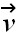(blue arrow) of the flow with respect to area vector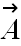(red arrow) of the window (surface).

Area is defined as a vector with its direction perpendicular to the surface (for a closed surface, the direction is always pointing outward).

The flux Φ through the window is defined as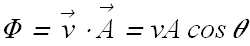Flux of an electric field

Flux Φ of Electric field through a plane surface of area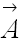is defined as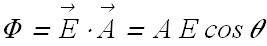If the area is of arbitrary shape, we can divide the area into small squares of area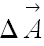.

The flux Φ of electric field through such an arbitrary area can be given as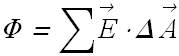Each square should be small enough so that we can neglect the curvature.

The direction of each area vector should be normal to the surface pointing outwards.

Dot product is a scalar product so flux is a scalar quantity.

When area vector approach a definitive limit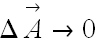, the summation becomes integral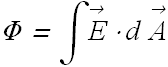Checkpoint 1 (Flux of Electric Field)

The figure here shows a cube of face area A immersed in a uniform electric E field that has the positive direction of the z-axis.
In terms of E and A, what is the flux through
(a) the front face (which is in the xy plane),
(b) the rear face,
(c) the top face, and (d) the whole cube?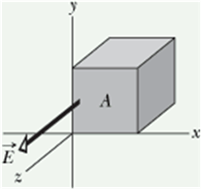Solution

Problem 1 (Flux of Electric Field)

A nonuniform electric field given bypierces the cube of edge 2.0 m shown in the figure (E is in newtons per coulomb and x is in meters.) What is the electric flux through the right face, the left face, and the top face?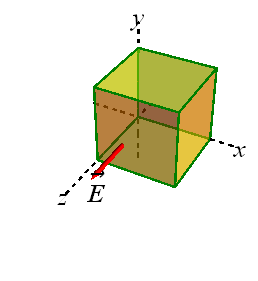Solution

Why we want Gauss’ Law?

Computing the electric field due to a distribution of charge is an involved job.

Gauss law makes those calculations extremely simple in many situations (not all situations)

Gauss’ law relates the electric field flux through a closed surface to the net charged enclosed by the surface.

A closed surface is also called a Gaussian surface. It can have any arbitrary shape.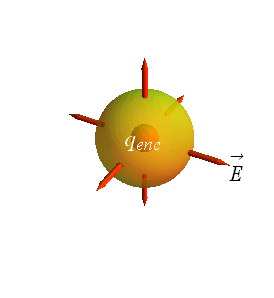Gauss’ Law

Gauss’ law relates net flux Φ of an electric field through a closed surface (a Gaussian surface) to the net charge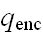that is enclosed in that surface.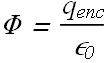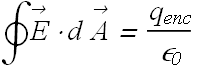This hold only when the net charge is located in a vacuum or in air (approximately air can be treated as vacuum).is the algebraic sum of all the positive and negative charges. Therefore it can have any value, positive, negative or zero.

Whenis positive the net flux is outward.

When theis negative the net flux is inward.

Charge outside the surface is not included in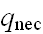, no matter how close is that to the surface.

The “shape” of the surface enclosing the net charge “does not affect” the total flux as the net charge enclosed is constant

Checkpoint 2 (Gauss’ Law)

The figure shows three situations in which a Gaussian cube sits in an electric field. The arrows and the values indicate the directions of the field lines and the magnitudes (in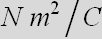) of the flux through the six sides of each cube. (The lighter arrows are for the hidden faces.)
In which situation does the cube enclose (a) a positive net charge, (b) a negative net charge, and (c) zero net charge?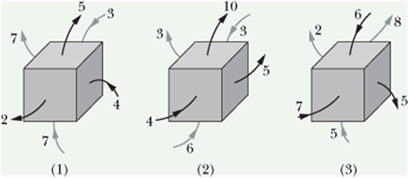Solution

Gauss’ Law and Coulomb’s Law

For a charge q placed at the center of a spherical surface, Gauss’ law relates net flux Φ of an electric field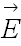as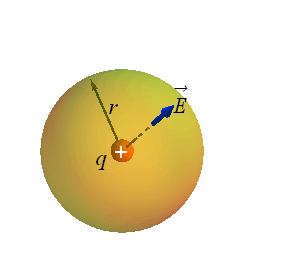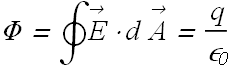Distance of each point on the sphere surface from the center is same therefore we can assume that the magnitude of electric fieldon all the point on the surface is same.

Area vectoris always parallel to, therefore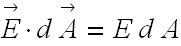.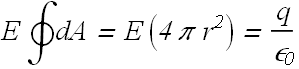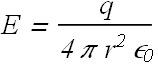Checkpoint 3 (Gauss’ Law and Coulomb’s Law)

There is a certain net flux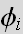through a Gaussian sphere of radius r enclosing an isolated charged particle.
Suppose the enclosing Gaussian surface is changed to
(a) a larger Gaussian sphere,
(b) a Gaussian cube with edge length equal to r, and
(c) a Gaussian cube with edge length equal to 2r.
In each case, is the net flux through the new Gaussian surface greater than, less than, or equal to?

Problem 2 (Gauss' Law)

A point charge of 1.8 μC is at the center of a cubical Gaussian surface 55 cm on edge. What is the net electric flux through the surface?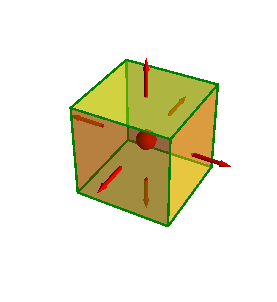Solution

What happens when a conductor is placed inside an electric field?

Consider a conductor placed in a uniform Electric field.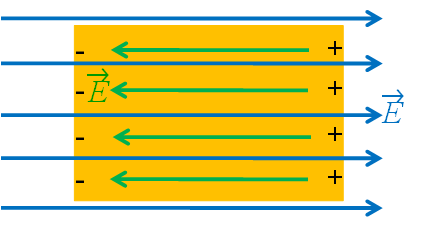The mobile charges (electrons) in the conductor will move opposite to the field.

This redistribution of charges will produce an induced field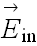inside the conductor.

Fieldwill be equal in magnitude to applied fieldbut opposite in direction.

Therefore net electric field inside the conductor will be zero.

Net electric field inside a conductor is always zero.

Direction of Field due to a uniformly charged linear source

Consider a uniformly charged very long linear source.

Consider a point P at distance much smaller than the length of the linear source.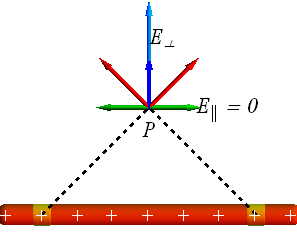Figure clearly shows that at point P parallel components (green) of electric field due to all the contributing pieces of linear source will cancel out therefore net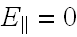.

Net field at point P will be sum of perpendicular (blue) components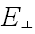of all the contributing pieces of linear source.

Electric field for a uniformly charged very long linear source is always normal to the linear source.

There is no field parallel to a uniformly charged very long linear source.

Same argument is true for an infinite uniformly charged sheet.

Applying Gauss’ law: Cylindrical Symmetry

Consider a cylindrical Gaussian surface with uniformly charged linear source along its axis.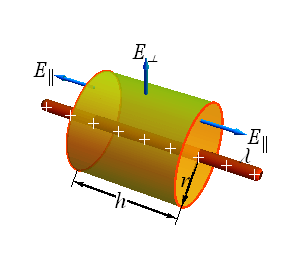According to Gauss' Law, net flux Φ through Gaussian cylinder is related to enclosed charge.Net flux Φ of an electric field in the cylinder will be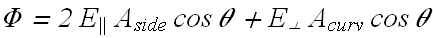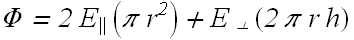For an infinite long sourceand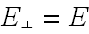, therefore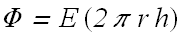If λ is the linear density of charge, total charge enclosedwill be given as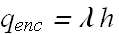According to Gauss' Law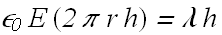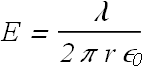Applying Gauss’ law: Planar Symmetry

Consider a Gaussian cylinder intersecting the uniformly charged sheet.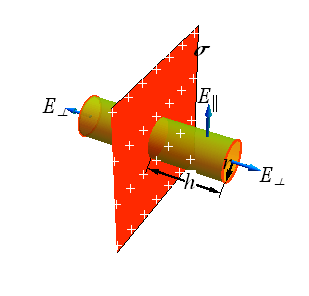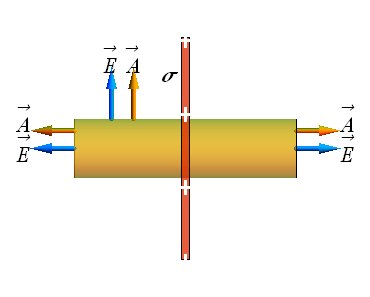According to Gauss' Law, net flux Φ through Gaussian cylinder is related to enclosed charge.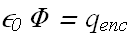Net flux Φ of an electric field in the cylinder will be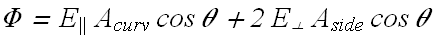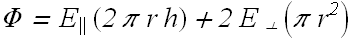For an infinite long sourceand, therefore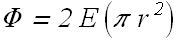If σ is the surface charge density, total charge enclosedwill be given as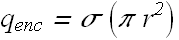According to Gauss' Law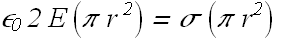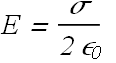It is independent of distance from the sheet

Electric field due to a uniformly charged Spherical Shell

Consider a uniformly charged shell (cross section is shown) of radius R (red shell) with total charge Q.When a spherical Gaussian surfaceof radius r<R is inside the shell, enclosed charge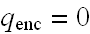, therefore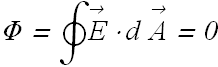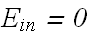When a spherical Gaussian surface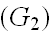of radius r>R is out side the shell, enclosed charge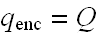.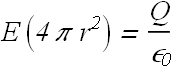Electric field due to a uniformly charged solid sphere

Consider a uniformly charged solid sphere (red) of radius R with total charge Q.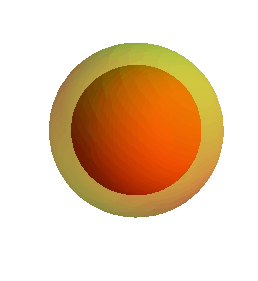Consider a spherical Gaussian surface of radius r>R out side the red sphere, the enclosed charge.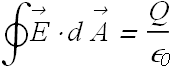Consider a spherical Gaussian surface of radius r<R inside the red sphere, the enclosed chargeis a fraction of total charge Q.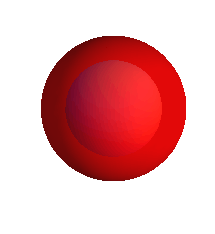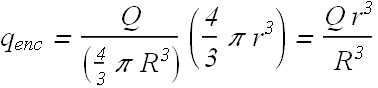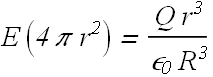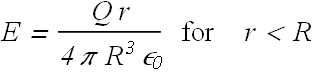Checkpoint 4 (Gauss’ Law and Coulomb’s Law)

The figure shows two large, parallel, non-conducting sheets with identical (positive) uniform surface charge densities, and a sphere with a uniform (positive) volume charge density.
Rank the four numbered points according to the magnitude of the net electric field there, greatest first.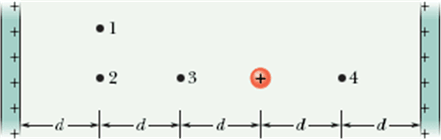Solution:

Both the infinite sheets produce uniform electric fields equal in magnitude but an opposite in direction.

Therefore the contribution to net electric field at any point due to the sheets will be “zero”.

The field experienced at different points is only due to the positively charged sphere.

Therefore the field at 3 and 4 will equal and maximum (closer to the sphere)
point 2 will be next in magnitude and point 1 will be last (farthest from center of sphere)